Home
IT Knowledge
Inspiration
Languages
EN

# MySQL - JOIN query cases with examples

13 points
Created by: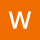Waleed-Gates
322

In this short article, we would like to show how to built MySQL JOIN queries for cases.

VisualisationSQL Query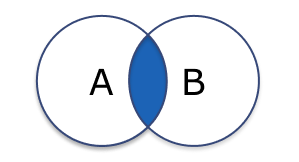INNER JOIN

``````SELECT *
FROM table_a A
JOIN table_b B ON A.key = B.key``````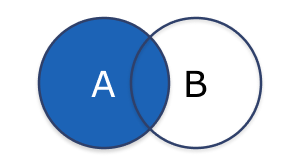LEFT OUTER JOIN

``````SELECT *
FROM table_a A
LEFT JOIN table_b B ON A.key = B.key``````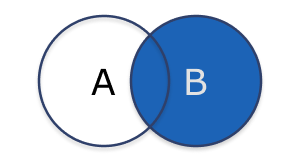RIGHT OUTER JOIN

``````SELECT *
FROM table_a A
RIGHT JOIN table_b B ON A.key = B.key``````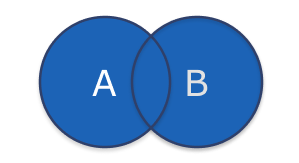FULL OUTER JOIN

``````SELECT * FROM table_a A
LEFT JOIN table_b B ON A.key = B.key
UNION
SELECT * FROM table_a A
RIGHT JOIN table_b B ON A.key = B.key``````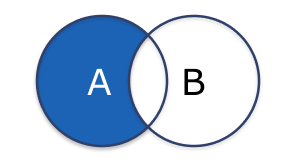LEFT OUTER JOIN with exclusion

``````SELECT  *
FROM table_a A
LEFT JOIN table_b B ON A.key = B.key
WHERE B.key IS NULL``````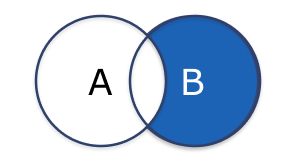RIGHT OUTER JOIN with exclusion

``````SELECT *
FROM table_a A
RIGHT JOIN table_b B ON A.key = B.key
WHERE A.key IS NULL``````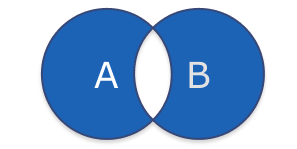FULL OUTER JOIN with exclusion

``````SELECT * FROM table_a A
LEFT JOIN table_b B ON A.key = B.key
WHERE B.key IS NULL
UNION
SELECT * FROM table_a A
RIGHT JOIN table_b B ON A.key = B.key
WHERE A.key IS NULL``````